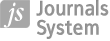ORIGINAL PAPER
Computational scheme for a differential difference equation with a large delay in convection term
,

More details
Hide details
1
Department of Mathematics, University College of Science, Osmania University, Hyderabad, Telangana, 500007, INDIA

Online publication date: 2023-06-28

Publication date: 2023-06-28

International Journal of Applied Mechanics and Engineering 2023;28(2):34–48

KEYWORDS
ABSTRACT
A computational scheme for the solution of layer behaviour differential equation involving a large delay in the derivative term is devised using numerical integration. If the delay is greater than the perturbation parameter, the layer structure of the solution is no longer preserved, and the solution oscillates. A numerical method is devised with the support of a specific kind of mesh in order to reduce the error and regulate the layered structure of the solution with a fitting parameter. The scheme is discussed for convergence. The maximum errors in the solution are tabulated and compared to other methods in the literature to verify the accuracy of the numerical method. Using this specific kind of mesh with and without the fitting parameter, we also studied the layer and oscillatory behavior of the solution with a large delay.

REFERENCES (26)
1.
Derstine M., Gibbs H., Hopf F.A. and Kaplan. D.L. (1982): Bifurcation gap in a hybrid optically bistable system.– Physical Review A, vol.26, No.6, pp.3720-3722.

2.
Kuang Y. (1993): Delay Differential Equations with Applications in Population Dynamics.– New York: Academic Press.

3.
Mackey M.C. and Glass. L. (1977): Oscillation and chaos in physiological control systems.– Science, vol.197, No.4300, pp.287-289.

4.
Stein R.B. (1967): Some models of neuronal variability.– Biophys. J., vol.7, No.1, pp.37-68.

5.
Bellman R., Cooke K. L. and Gillis J. (1963): Differential Difference Equations.– New York: Academic Press.

6.
Driver R.D. (1977): Ordinary and Delay Differential Equations.– New York: Springer.

7.
El'sgol'ts L.E. and Norkin S.B. (1973): Introduction to the Theory and Application of Differential Equations with Deviating Arguments.– New York: Academic Press.

8.
Smith H. (2011): An Introduction to Delay Differential Equations with Applications to the Life Sciences.– Berlin: Springer.

9.
Doolan E.P., Miller J.J. H. and Schilders W.H.A. (1980): Uniform Numerical Methods for Problems with Initial and Boundary Layers.– Dublin: Boole Press.

10.
Kokotovic P.V., Khalil H.K. and O’Reilly. J. (1986): Singular perturbation methods in control analysis and design. – New York: Academic Press.

11.
Miller J.J., O'Riordan E., Shishkin G.I. and Kellogg R.B. (1996): Fitted Numerical Methods for Singular Perturbation Problems.– Singapore: World Scientific.

12.
O’Malley R.E. (1974): Introduction to Singular Perturbations.– New York: Academic Press.

13.
Kadalbajoo M. K. and Sharma K. K. (2004): Numerical analysis of singularly perturbed delay differential equations with layer behavior.– Applied Mathematics and Computation, vol.157, No.1, pp.11-28.

14.
Kadalbajoo M.K. and Sharma K.K. (2008): A numerical method based on finite difference for boundary value problems for singularly perturbed delay differential equations.– Applied Mathematics and Computation, vol.197, No.2, pp.692-707.

15.
Kadalbajoo M.K. and Kumar D. (2008): Fitted mesh B-spline collocation method for singularly perturbed differential-difference equations with small delay.– Applied Mathematics and Computation, vol.204, No.1, pp.90-98.

16.
Lange C.G. and Miura R.M. (1994): Singular perturbation analysis of boundary-value problems for differential-difference equations. v. small shifts with layer behavior.– SIAM Journal on Applied Mathematics, vol.54, No.1, pp.249-272.

17.
Lange C.G. and Miura R.M. (1994): Singular perturbation analysis of boundary-value problems for differential-difference equations. vi. Small shifts with rapid oscillations.– SIAM Journal on Applied Mathematics, vol.54, No.1, pp. 273-283.

18.
Bansal K. and Sharma K.K. (2017): Parameter uniform numerical scheme for time dependent singularly perturbed convection diffusion-reaction problems with general shift arguments.– Numerical Algorithms, vol.75, No.1, pp.113-145.

19.
Das P. (2019): An a posteriori-based convergence analysis for a nonlinear singularly perturbed system of delay differential equations on an adaptive mesh.– Numerical Algorithms, vol.81, No.2, pp.465-487.

20.
Ravi Kanth A.S.V. and Murali Mohan Kumar P. (2020): Computational results and analysis for a class of linear and nonlinear singularly perturbed convection delay problems on Shishkin mesh.– Hacettepe Journal of Mathematics & Statistics, vol.49, No.1, pp. 221-235.

21.
Ravi Kanth A.S.V. and Murali P. (2017): Numerical treatment for a singularly perturbed convection delayed dominated diffusion equation via tension splines.– International Journal of Pure and Applied Mathematics, vol.6, No.113, pp.110-118.

22.
Kumara Swamy D., Phaneendra K. and Reddy Y.N. (2018): Accurate numerical method for singularly perturbed differential-difference equations with mixed shifts.– Khayyam J. Math., vol.4, No.2, pp.110-122.

23.
Lalu M., Phaneendra K. and Emineni S.P. (2021): Numerical approach for differential-difference equations having layer behaviour with small or large delay using non-polynomial spline.– Soft Computing, vol.25, No.21, pp.13709-13722.

24.
Habtamu Garoma Debela and Gemechis File Duressa (2020): Accelerated fitted operator finite difference method for singularly perturbed delay differential equations with non-local boundary condition.– Journal of the Egyptian Mathematical Society, vol.28, No.16, pp.1-16.

25.
Varga R.S. (1962): Matrix Iterative Analysis.– New Jersey: Prentice-Hall.

26.
Young D.M. (1971): Iterative Solutions of Large Linear Systems.– New York: Academic Press.

 eISSN: 2353-9003 ISSN: 1734-4492